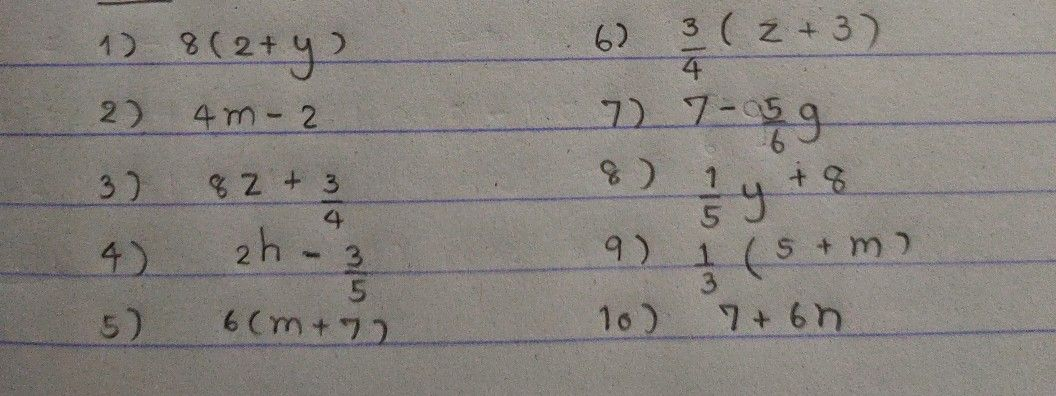เครื่องหมาย
โจทย์ปัญหาTranslate Algebraic Expressions $1\right)$ 8 times the sum of 2 and y $2\right)$ 2 less than 4 times m $3\right)$ Add $three-f0∪nhg$ to 8 times z $4\right)$ Subtract $1hree-ti\pi hs$ from 2 times h $5\right)$ 6 times the sum of m and 7 $6\right)$ $Three-tounhs$ of the sum of z and 3 $7\right)$ $Five-si\times ths$ of g is subtracted from 7 $8\right)$ 8 is added to $One-ti\pi h$ of y $9\right)$ $One-third$ of the sum of 5 and m $10\right)$ $Ad0$ $7t0$ 6 times n
มัธยมต้น
อังกฤษ
เนื้อหาคำถาม
แปลอันนี้ให้หน่อยครับ
วิธีการแก้ปัญหาคุณครู Qanda - Jiยังไม่เข้าใจใช่ไหม?
ถามคำถามกับครู Qanda